Home | Contact | Sitemap
Call us: +51 84 241424

# Promotion Belmond luxery hotels Lima, Cusco, Sacred valley and Machu picchu + Colca canyon Peru June, July, August 2018

[Sassy_Social_Share style="background-color:transparent;"]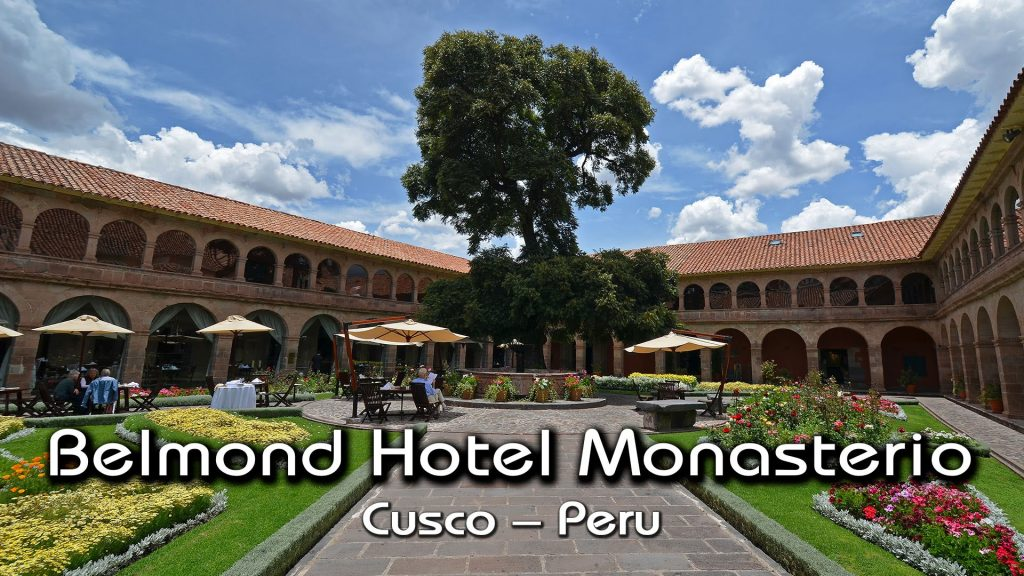August + Septiembre |

The luxery hotel chain Belmond

Special promotion only en special days !

## 1 BELMOND miraflores Park hotel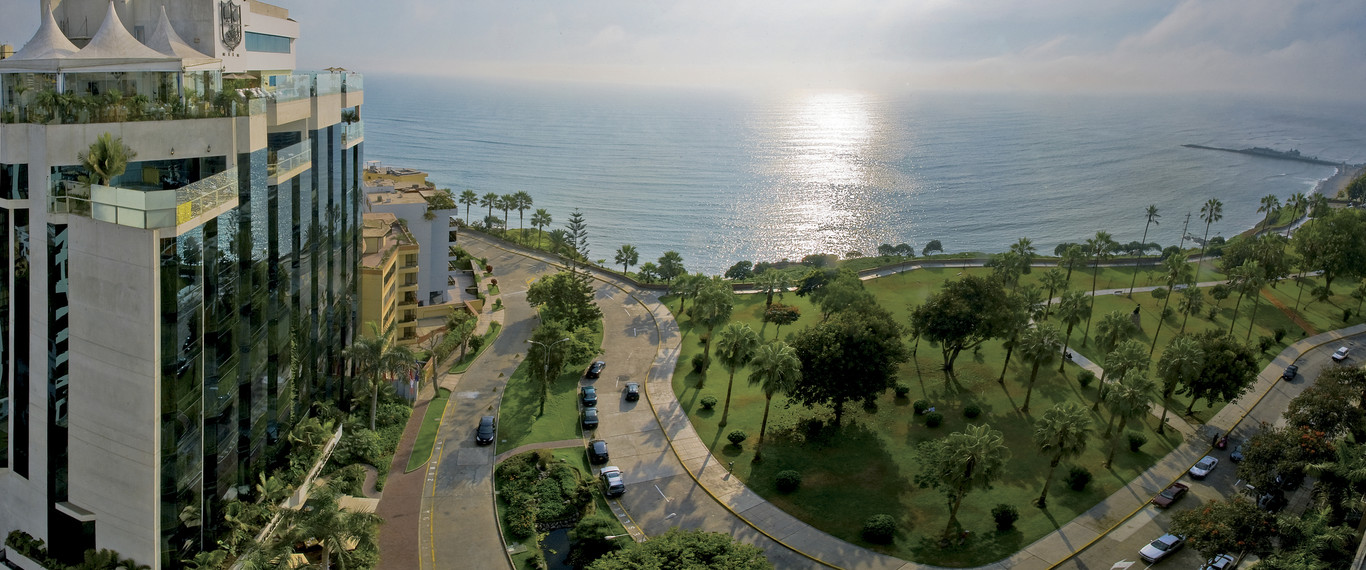July 7+8+9+10+11+12+13+14+15+17+18+26+30+31

August 1+14+15+16+19+21+23

September 7+9+10+11+12+13+16+17+18+20+21+24+25+26+27+30

Special price = city view junior suit  = 335 usd a double room  Normal price  855 USD
Special price = Ocian view junior suit  = 373 usd a double room  Normal price  905 USD

## 2 BELMOND hotel Monasterio Cusco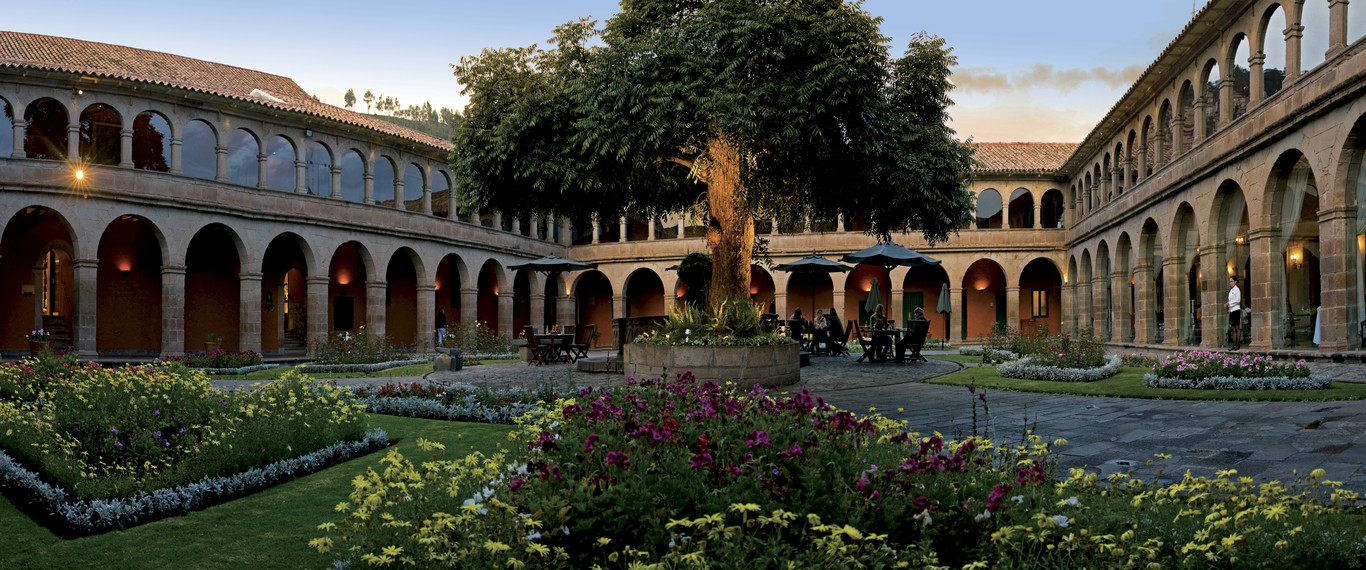July 13+14+17+18+20+21+22+23+24+25+27+29+30+31

August 3+4+5 + 17+18+20+21+22+27+28+30+31

September 1+2+3+4+11+12+13+14+15+16+17+18+19+20+21+29

Special price = superior room = 249 usd a double room  Normal price  760 USD
Special price = deluxe room  = 279 usd a double room  Normal price  845 USD

## 3 BELMOND hotel Nazarenas Cusco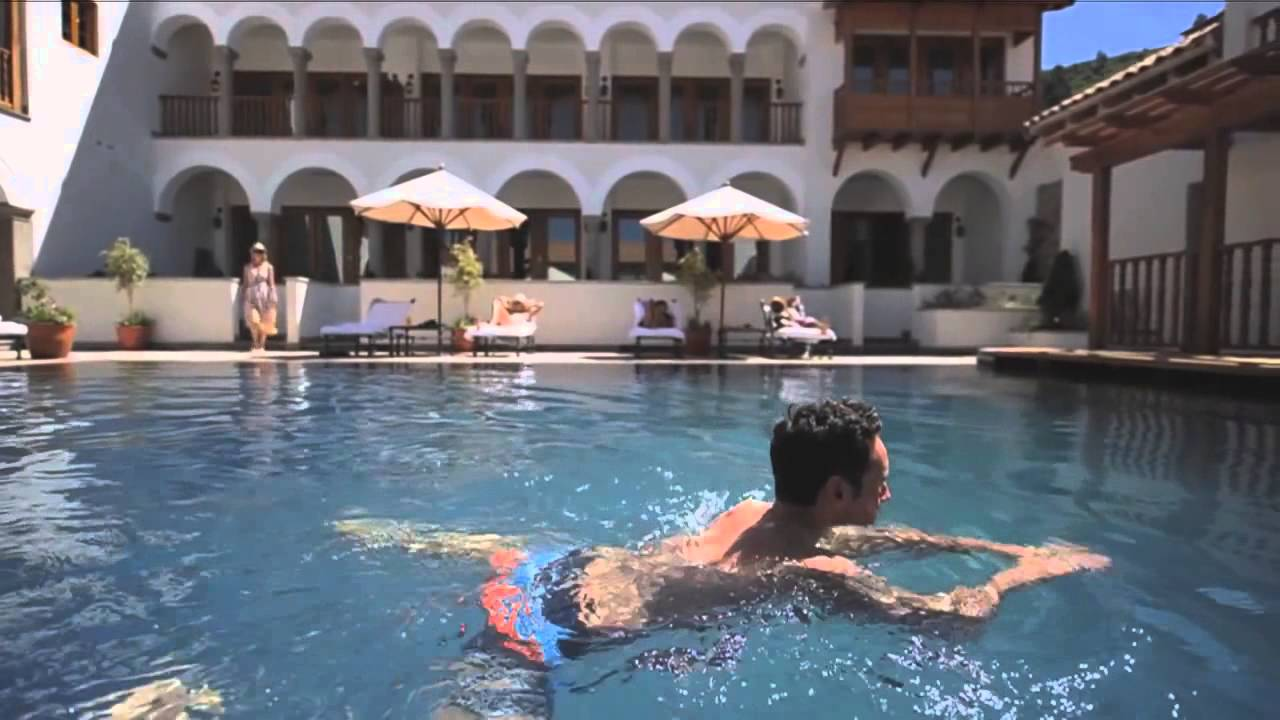July 7+8+9+11+12+13+14+15+16+17+18+19+20+21+22+27+28+29+30

August 1+3+4+6 +12+18+19+20+24+25+26+27

September 2+3+4+5+6+7+8+9+10+11+15+16+17+18+22+23+24+25+26+27

Special price = junior suit  = 399 usd a  room Normal price  995  USD

## 4 BELMOND hotel rio sagrado Sacred valley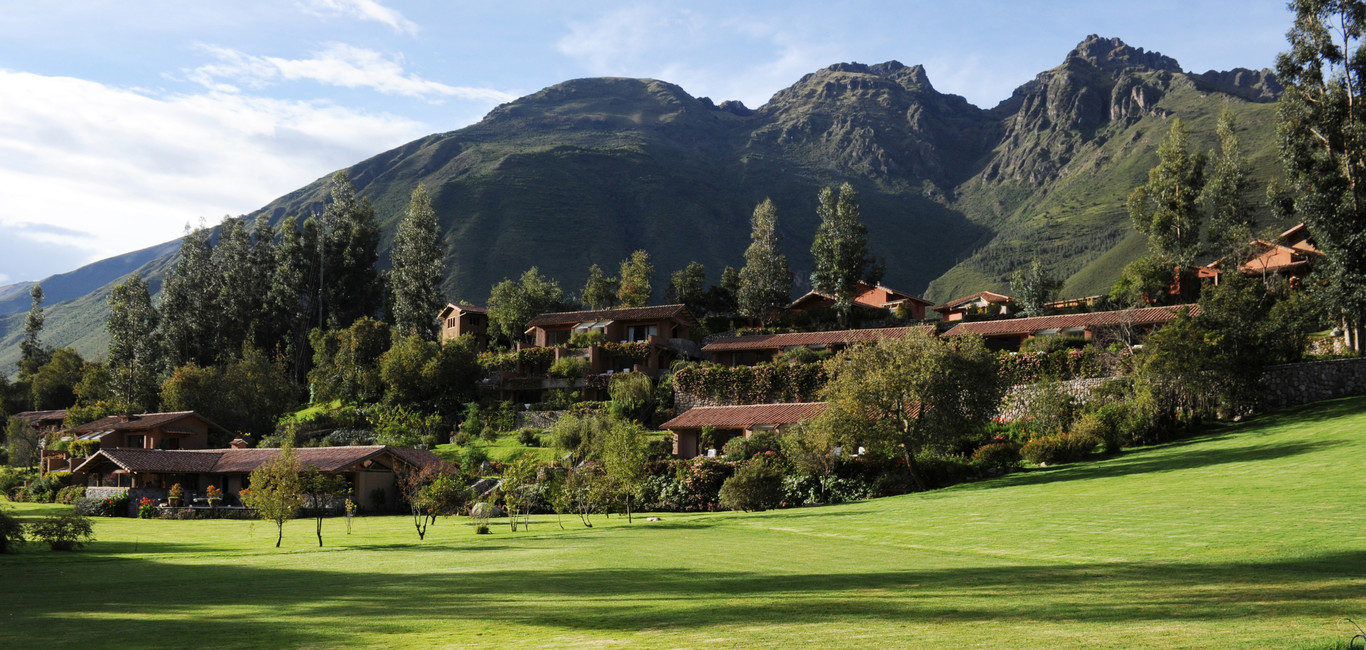August 28+31

September 2+19+22+23+26

Special price = deluxe terrace  room = 249 usd a double room  Normal price  630 USD
Special price = garden junior suit = 275 usd a double room  Normal price  680 USD

## 5 BELMOND hotel santuario lodge / At machu picchu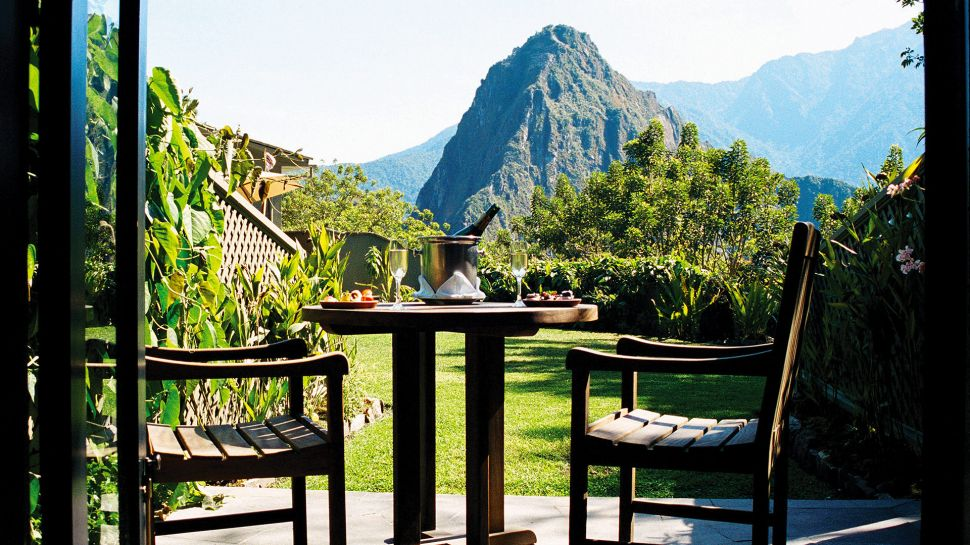NO PROMOTION IN JULY / AUGUST/ SEPTEMBER

Special price only Bed & breakfast = standard room = 545 usd a double room  Normal price  1400 USD
Special price only Bed & breakfast = standard patio = 605 usd a double room  Normal price  1500 USD

## 6 BELMOND hotel las casitas / At colca canyonJuly  1+2+3 +4+5+6+7+8+9+10+11+12+13+14+16+17+18+19+21+22+23+28+29+30+31

August 1+6+9  11+12+13+15+16+17+18+19+20+21+22+23+24+27+28+29+30+31

September 1+2+3+4+5+6+7+8+11+12+13+14+15+23+24+25+27+28+29+30

October  1+2+3+4+5+8+9+10+11+12+13+14+16+17+18+19+20+21+24+25+26+27+28+29+30+31

Special price  casita with garden view excluded diner = 330 usd a double room  Normal price  600 USD

## Promotion Belmond luxery hotels Lima, Cusco, Sacred valley and Machu picchu + Colca canyon Peru June, July, August 2018

Add personReserve Consult
 1° Person *Name* : *Surname* : *Country* : Date of birth : 01020304050607080910111213141516171819202122232425262728293031 JanuaryFebruaryMarchAprilMayJuneJulyAugustSeptemberOctoberNovemberDecember 193019311932193319341935193619371938193919401941194219431944194519461947194819491950195119521953195419551956195719581959196019611962196319641965196619671968196919701971197219731974197519761977197819791980198119821983198419851986198719881989199019911992199319941995199619971998199920002001200220032004200520062007200820092010201120122013201420152016 *Passport number* : Add passport : *Email* :